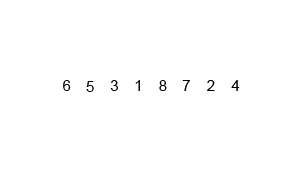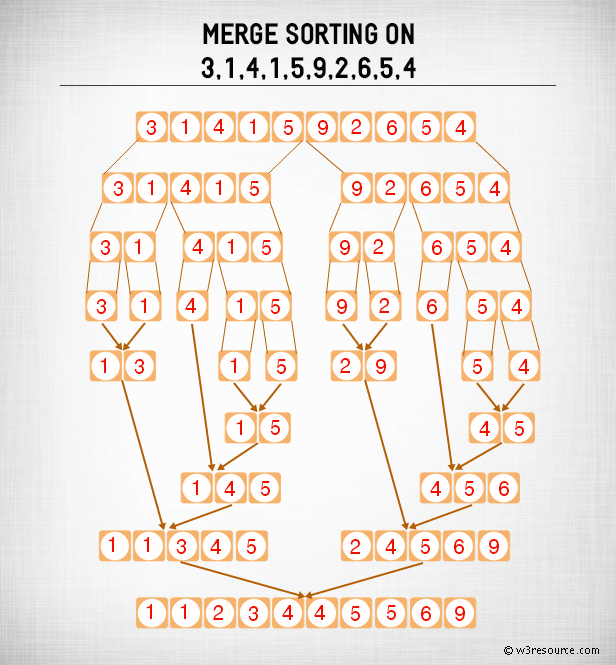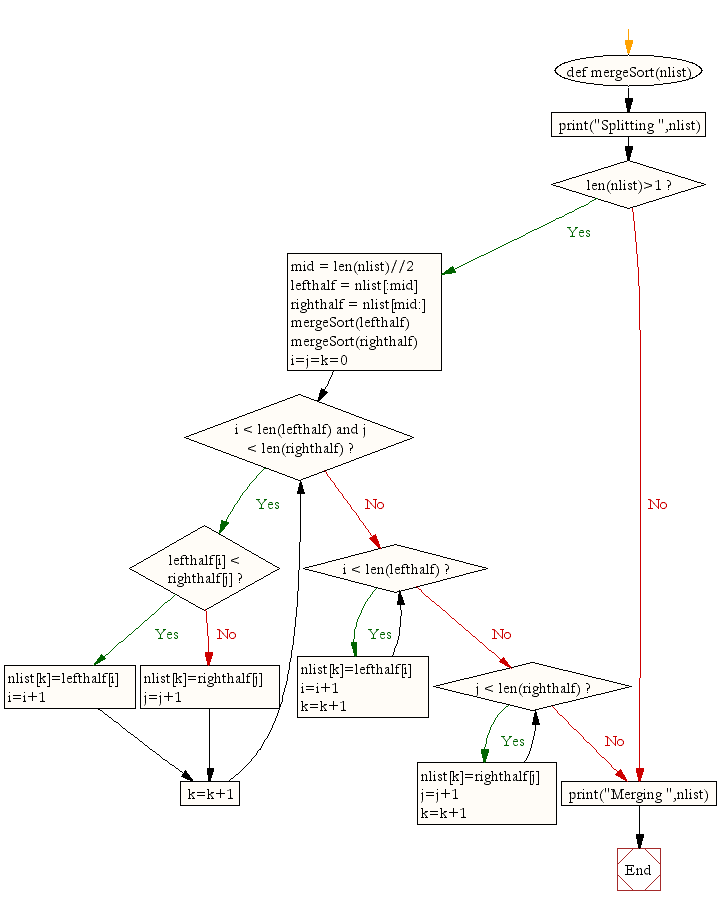﻿ Python: Merge sort - w3resource# Python: Merge sort

## Python Search and Sorting: Exercise-8 with Solution

Write a Python program to sort a list of elements using the merge sort algorithm.
Note: According to Wikipedia "Merge sort (also commonly spelled mergesort) is an O (n log n) comparison-based sorting algorithm. Most implementations produce a stable sort, which means that the implementation preserves the input order of equal elements in the sorted output."

Algorithm:

Conceptually, a merge sort works as follows :

• Divide the unsorted list into n sublists, each containing 1 element (a list of 1 element is considered sorted).
• Repeatedly merge sublists to produce new sorted sublists until there is only 1 sublist remaining. This will be the sorted list.

An example of merge sort:Merge Sort: Pictorial PresentationSample Solution:

Python Code:

``````def mergeSort(nlist):
print("Splitting ",nlist)
if len(nlist)>1:
mid = len(nlist)//2
lefthalf = nlist[:mid]
righthalf = nlist[mid:]

mergeSort(lefthalf)
mergeSort(righthalf)
i=j=k=0
while i < len(lefthalf) and j < len(righthalf):
if lefthalf[i] < righthalf[j]:
nlist[k]=lefthalf[i]
i=i+1
else:
nlist[k]=righthalf[j]
j=j+1
k=k+1

while i < len(lefthalf):
nlist[k]=lefthalf[i]
i=i+1
k=k+1

while j < len(righthalf):
nlist[k]=righthalf[j]
j=j+1
k=k+1
print("Merging ",nlist)

nlist = [14,46,43,27,57,41,45,21,70]
mergeSort(nlist)
print(nlist)
```
```

Sample Output:

```Splitting  [14, 46, 43, 27, 57, 41, 45, 21, 70]
Splitting  [14, 46, 43, 27]
Splitting  [14, 46]
Splitting  
Merging  
Splitting  
-------
Merging  [14, 21, 27, 41, 43, 45, 46, 57, 70]
[14, 21, 27, 41, 43, 45, 46, 57, 70]
```

Flowchart:## Visualize Python code execution:

The following tool visualize what the computer is doing step-by-step as it executes the said program:

Python Code Editor :

What is the difficulty level of this exercise?

Test your Programming skills with w3resource's quiz.

﻿

## Python: Tips of the Day

The Zip() Function:

```>>> students = ('John', 'Mary', 'Mike')
>>> ages = (15, 17, 16)
>>> scores = (90, 88, 82, 17, 14)
>>> for student, age, score in zip(students, ages, scores):
...     print(f'{student}, age: {age}, score: {score}')
...
John, age: 15, score: 90
Mary, age: 17, score: 88
Mike, age: 16, score: 82
>>> zipped = zip(students, ages, scores)
>>> a, b, c = zip(*zipped)
>>> print(b)
(15, 17, 16)
```